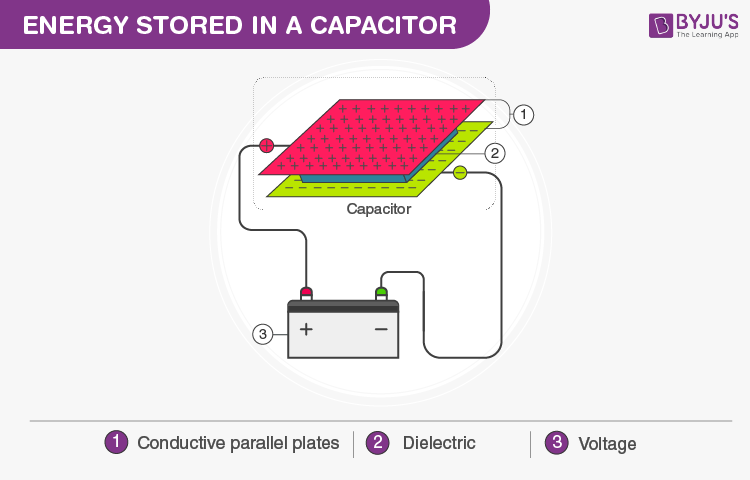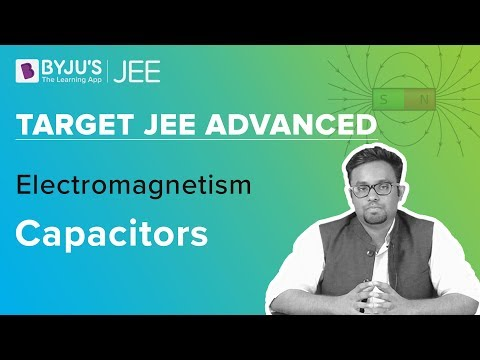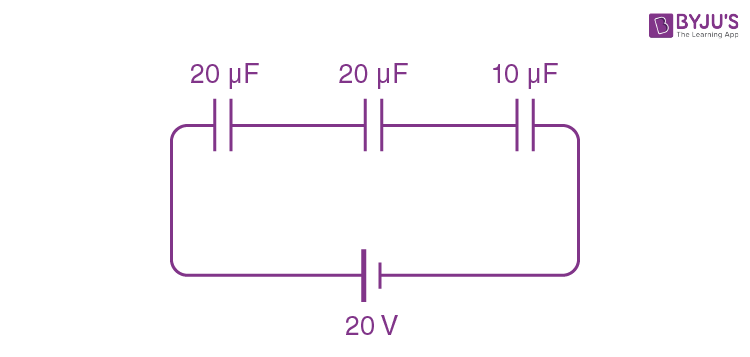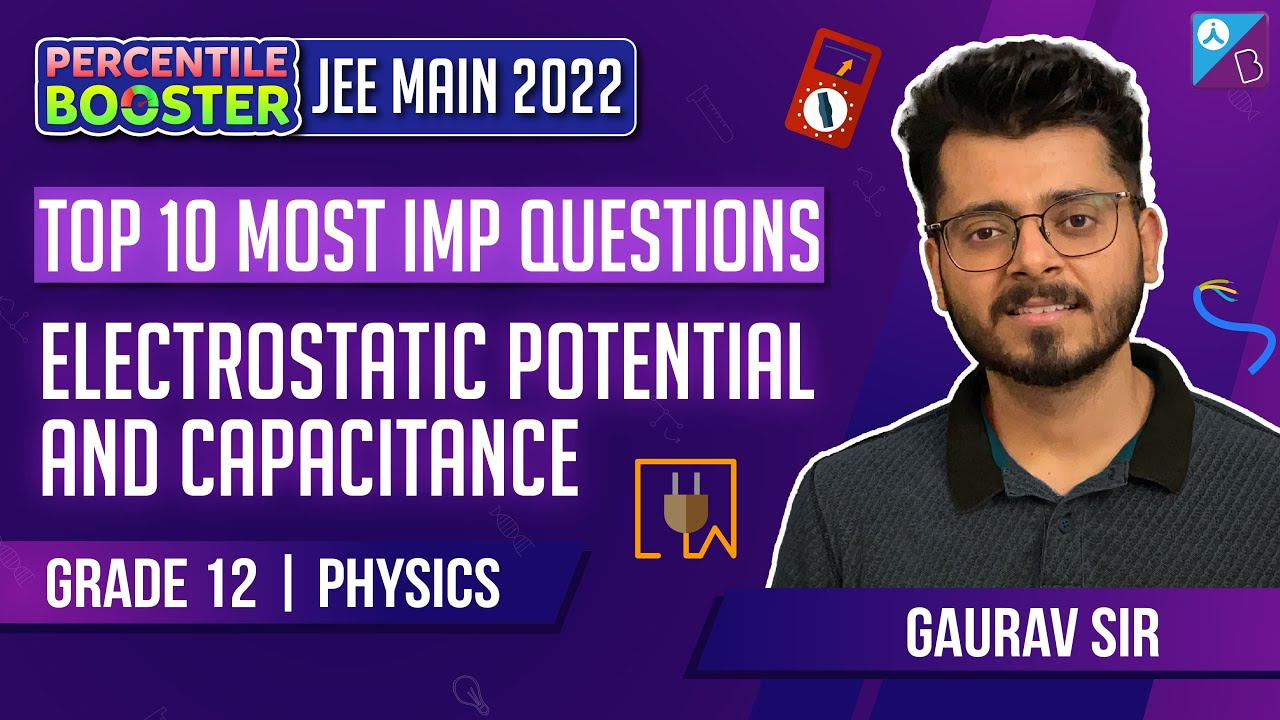Win up to 100% scholarship on Aakash BYJU'S JEE/NEET courses with ABNAT Win up to 100% scholarship on Aakash BYJU'S JEE/NEET courses with ABNAT

# Energy Stored by Capacitor

We know that a capacitor is used to store energy. In this module, we will discuss how much energy can be stored in a capacitor, the parameters that the energy stored depends upon and their relations.

## How to Calculate the Energy Stored in Capacitor?

Work has to be done to transfer charges onto a conductor against the force of repulsion from the already existing charges on it. This work done to charge from one plate to the other is stored as the potential energy of the electric field of the conductor.C = Q/V

Suppose the charge is being transferred from plate B to A. At the moment, the charge on the plates is Q’ and –Q’. Then, to transfer a charge of dQ’ from B to A, the work done by an external force will be

$$\begin{array}{l}dW=VdQ’=\frac{Q’}{C}dQ'\end{array}$$

Total work done =

$$\begin{array}{l}\,\int\limits_{0}^{O}{\frac{1}{C}Q’dQ’=\frac{{{Q}^{2}}}{2C}}\end{array}$$

∴ Energy stored in a capacitor

$$\begin{array}{l}=\frac{{{Q}^{2}}}{2C}=\frac{1}{2}C{{V}^{2}}=\frac{1}{2}QV\end{array}$$

### Watch This Video for More Details### Energy Density in an Electric Field

Energy stored per unit volume is called energy density. It is given by

$$\begin{array}{l}U = \frac{1}{2}{{\varepsilon }_{0}}{{E}^{2}}\end{array}$$

$$\begin{array}{l}, i.e., U = \frac{1}{2}k\varepsilon {{E}^{2}}\end{array}$$
with a dielectric of dielectric constant k is introduced, and E is the net electric field in the dielectric medium.

## Problems on Energy Stored in a Capacitor

Problem 1: A battery of 20 V is connected to 3 capacitors in series, as shown in the figure. Two capacitors are of 20μF each, and one is of 10μF. Calculate the energy stored in the capacitors in the steady state.Sol:

$$\begin{array}{l}\frac{1}{{{C}_{eff}}}=\frac{1}{20}+\frac{1}{20}+\frac{1}{10}=\frac{4}{20}=\frac{1}{5}\end{array}$$

Ceff = 5μF

The energy stored

$$\begin{array}{l}=\frac{1}{2}C{{V}^{2}}=\frac{1}{2}\times 5\times {{10}^{-6}}\times {{20}^{2}}={{10}^{-3}}J\end{array}$$

Problem 2: A parallel plate capacitor has plates of an area of 4 m2 separated by a distance of 0.5 mm. The capacitor is connected across a cell of emf 100 volts. Find the capacitance, charge and energy stored in the capacitor if a dielectric slab of dielectric constant k = 3 and thickness 0.5 mm is inserted inside this capacitor after it has been disconnected from the cell.

Sol: When the capacitor is without dielectric

$$\begin{array}{l}{{C}_{0}}=\frac{{{\varepsilon }_{0}}A}{d}=\frac{8.85\times {{10}^{-12}}\times 4}{0.5\times {{10}^{-3}}}\end{array}$$

$$\begin{array}{l}{{C}_{0}}=7.08\times {{10}^{-2}}\mu F.\end{array}$$

$$\begin{array}{l}{{Q}_{0}}={{C}_{0}}{{V}_{0}}\end{array}$$

$$\begin{array}{l}={{(7.08\times {{10}^{-2}}\times 100)}_{\mu C}}=7.08\mu C\,\end{array}$$

$$\begin{array}{l}{{U}_{0}}=\frac{1}{2}{{C}_{0}}V_{0}^{2}=354\times {{10}^{-6}}J\end{array}$$
as the cell has been disconnected, and the charge on the capacitor remains constant.

$$\begin{array}{l}C = \frac{k{{\varepsilon }_{0}}A}{d}=k{{C}_{0}}=0.2124\mu F\end{array}$$

$$\begin{array}{l}V = \frac{Q}{C}=\frac{{{Q}_{0}}}{k{{C}_{0}}}\frac{{{V}_{0}}}{k}=\frac{100}{3}\ \text{volts}\end{array}$$

$$\begin{array}{l}U = \frac{1}{2}\frac{{{Q}_{0}}}{C}=\frac{1}{2}\frac{Q_{0}^{2}}{k{{C}_{0}}}\frac{{{U}_{0}}}{k}=118\times {{10}^{-6}}J\end{array}$$

### Electrostatic Potential and Capacitance## Frequently Asked Questions on Energy Stored in Capacitor

Q1

### Does energy get stored in a capacitor?

Yes, in a capacitor, the energy gets stored in the space between the two plates.

Q2

### What type of energy is stored in a capacitor?

Electrostatic potential energy gets stored in the capacitor. It is, thus, related to the charge and voltage between the plates of the capacitor.

Q3

### Where does the energy stored in a capacitor reside?

When a charged capacitor is disconnected from a battery, its energy remains in the field in the space between its plates.

Q4

### How to increase the energy stored in a capacitor?

The energy of the capacitor depends on the capacitance and the voltage of the capacitor. If the capacitance, voltage or both are increased, the energy stored by the capacitor will also increase. A dielectric slab can be added between the plates of the capacitor to increase the capacitance of the capacitor.

Test your Knowledge on energy stored in capacitor